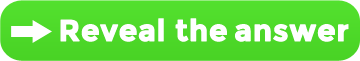# You’re A Math Genius If You Solve This Tricky Mathematical Brainteaser

In this math puzzle there are four numbers given in each square which relate to each other with mathematical relationships. All you have to do is find the pattern and then find the missing number:Did you figure it out? Click below to check the answer:SHARE this challenging brainteaser to test your friends and family!